Courses

# Test Level 1: Relations And Functions- 1

## 25 Questions MCQ Test Mathematics For JEE | Test Level 1: Relations And Functions- 1

Description
This mock test of Test Level 1: Relations And Functions- 1 for JEE helps you for every JEE entrance exam. This contains 25 Multiple Choice Questions for JEE Test Level 1: Relations And Functions- 1 (mcq) to study with solutions a complete question bank. The solved questions answers in this Test Level 1: Relations And Functions- 1 quiz give you a good mix of easy questions and tough questions. JEE students definitely take this Test Level 1: Relations And Functions- 1 exercise for a better result in the exam. You can find other Test Level 1: Relations And Functions- 1 extra questions, long questions & short questions for JEE on EduRev as well by searching above.
QUESTION: 1

### The domain of the function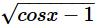is

Solution:

For f to be defined term under square root has to be greater than zero.
⇒ cosx − 1 ≥ 0
⇒ cosx ≥ 1
Only possible if cosx = 1
⇒ x = 2nπ ∀ n∈Z

QUESTION: 2

### The domain of the function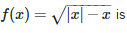Solution:

Since you want the square root, 1-cos(x) must not be negative.
Solve 1-cos(x) ≥ 0
cos(x) ≤ 1
But -1 ≤ cos(x) ≤ 1 for all "x".
So Domain is All Real Numbers.

QUESTION: 3

### The domain of the function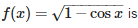Solution: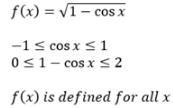QUESTION: 4

The domain of the function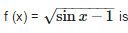Solution: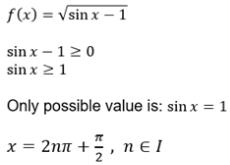QUESTION: 5

Let x be any real, then [x + y] = [x] + [y] holds for

Solution:

Let y has some fractional part
x = 6.5
y = 0.9
[x] = 6, [y] = 0, [x]+[y] = 6
[x + y] = [7.4] = 7
[x + y] ≠ [x]+[y]
Let x, y be integers
x = 6.5
y = 1
[x] = 6, [y] = 1, [x]+[y] = 7
[x + y] = [7.5] = 7
[x + y] = [x]+[y]
So, [x + y] = [x]+[y] holds for y ϵ I

QUESTION: 6

The function f (x) = x + cos x

Solution:

f(x) = x
g(x) = cosx
D(f) = R
D(g) = R
D(f+g) = D(f) ⋂ D(g)
So , D(f+g) = R
Therefore, given function is defined for real numbers.

QUESTION: 7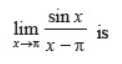Solution:

lim(x → π) sinx/x-π
lim(h → 0) sin(π-h)/π-h-π
lim(h → 0) -sinh/h
⇒ -1

QUESTION: 8

A function / from the set of natural numbers to integers defined by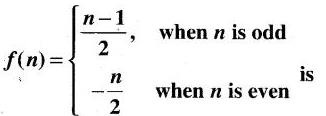Solution:

Clearly/is both one - one and onto Because if n is odd, values are set of all non-negative integers and if n is an even, values are set of all negative integers.

QUESTION: 9

If f (x) =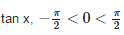and g (x) =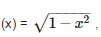then domain of fog is

Solution: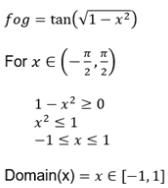QUESTION: 10

If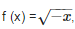then domain of fof is

Solution: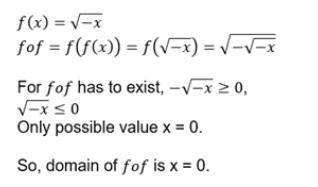QUESTION: 11

If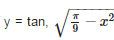then

Solution:
QUESTION: 12

The interval in which the values of f (x) lie where f (x) = 3 sin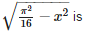Solution:

f(x) = 3.sin(√π2/16 − x2)
since the quantity within the square root can not be negative.
therefore
π2/16 − x2 ≥ 0
x2 ≤ π2/16
−π/4 ≤ x ≤ π/4
the minimum value of the function is 3.sin0=0
and the maximum value of the function is 3sinπ/4 = 3/√2
therefore range is [0, 3/√2]

QUESTION: 13

The range of the function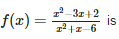Solution:

y = (x2 - 3x + 2)/(x2 + x -6)
= [(x-2)(x-1)]/[(x-2)(x+3)]
= (x-1)/(x+3)
= 1 - 4/(x+3)
x is not equal to 2 and -3
For x = 2, y = 1/5
for x = -3, y → 1
Range (y): R - {1, 1/5}

QUESTION: 14

If f(x) =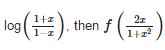is equal to

Solution:

f(x) = log[(1+x)/(1-x)]    ……….(1)
Substitute 2x/1+x2 in place of x in equation(1)
f(2x1+x2)=log⁡(1+2x1+x2)(1−2x1+x2)
⟹f(2x/(1+x2))=log[1+(2x/(1+x2))/(1-(2x/1+x2))]
⟹f(2x/(1+x2))=log⁡((1+x2+2x)/(1+x2−2x))
⟹f(2x/(1+x2))=log((1+x)2 / (1−x))2
⟹f(2x/ (1+x2))=log⁡(1+x / 1−x)2
We know that log⁡mn=nlog⁡m
⟹f(2x/(1+x2))=2log⁡(1+x / 1−x)
From equation (1):
f(2x/(1+x2))=2f(x)

QUESTION: 15

If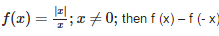is equal to

Solution:
QUESTION: 16

Which of the following functions is not one-one ?

Solution:
QUESTION: 17

If f (x)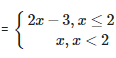then f (1) is equal to

Solution: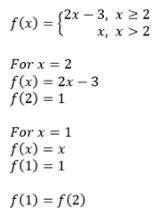QUESTION: 18

The domain of the real-valued function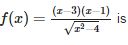Solution:

f(x) = (x-3)(x-1)/[(x2 -4)^1/2]
= x2 - 4 = 0
(x-2)(x+2) = 0
x = 2 and -2
(-∞, -2 ) U (2,∞)

QUESTION: 19

A condition for a function y = f (x) to have an inverse is that it should be

Solution:

For a function to have its inverse in a given domain, it should be continuous in that domain and should be a one-one function in that domain.
If the function is one-one in the domain, then it has to be strictly monotonic.
For example y=sin(x) has its domain in xϵ[−π/2,π/2] since it is strictly monotonic and continuous in that domain.

QUESTION: 20

Set A has 3 elements and set B has 4 elements. The number of injections that can be defined from A to B is

Solution:

Given that n(A)=3 and n(B)=4, the number of injections or one-one mapping is given by.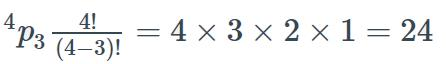QUESTION: 21

The range of the function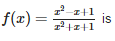Solution:

yx​2 + xy + y = x2 - x + 1
(y-1)x2 + (y+1)x + y-1 = 0
if y = -1
-2x2 -1 -1 = 0
-2x2 -2 = 0
Therefore, y cannot be equal to -1 since x will be a complex value
Now, y not equal to -1.
(y-1)x2 + (y+1)x + y-1 = 0   has real roots
hence, (y+1)2 - 4 (y-1)(y-1) ≥ 0
y2 + 2y + 1 - 4y2 + 8y - 4 ≥ 0
-3y2 + 10y - 3 ≥ 0
3y2 - 10y + 3 ≤ 0
3y2 - 9y - y + 3 ≤ 0
3y (y-3) - 1 (y-3) ≤0
(3y-1)(y-3) ≤ 0
y ∈ [1/3,3]
Hence, range of function is [1/3,3]

QUESTION: 22

On the set Z of all integers define f ; Z → Z as follows : f (x) = x/2 if x is even, and f (x) = 0 if x is odd , then f is

Solution:

f(x) = {x/2 x is even,     0 x is odd}
f(1) = f(3) = f(5) = f(odd) = 0
Hence f is not one - one.
column = Z
Range = {0, even}
= {0,2,4,6,8,10.......}
f(x) = x/2 implies even
f(odd) = 0
Range is not equal to codomain
Hence, onto

QUESTION: 23

Let f : R → R be defined by f (x) = 3x – 4, then f-1 (x) is equal to

Solution:

f(x) = 3x - 4
Let f(x) = y
y = 3x - 4
y + 4 = 3x
x = (y+4)/3
x → f-1(x) and y → x
f-1(x) = (x+4)/3

QUESTION: 24

The number of bijective functions from the set A to itself when A constrains 106 elements is

Solution:

The number of bijections from A to A, will be n! for n elements is A.

Now there are 106, elements.

Hence the number of bijections will be n!=(106)!

QUESTION: 25

In the set W of whole numbers an equivalence relation R defined as follow : aRb iff both a and b leave same remainder when divided by 5. The equivalence class of 1 is given by

Solution:

aRb iff both a and b leave same remainder when divided by 5.
a= 5x+n
a-1= 5x
b=5y+n
b-1 =5y
Equivalence class = {1,6,11,16,21}